• 累计撰写 63 篇文章
• 累计创建 16 个标签
• 累计收到 5 条评论

### 目 录CONTENT# 经典排序算法总结2022-05-04 / 0 评论 / 0 点赞 / 100 阅读 / 5,996 字 / 正在检测是否收录...

### 1、冒泡排序

1.1 算法描述

• 比较相邻的元素。如果第一个比第二个大，就交换它们两个；
• 对每一对相邻元素作同样的工作，从开始第一对到结尾的最后一对，这样在最后的元素应该会是最大的数；
• 针对所有的元素重复以上的步骤，除了最后一个；
• 重复步骤 1~3，直到排序完成。

1.2 动图演示1.3 代码实现

 //冒泡排序
public static void  bubbleSort(int[] arr){

for(int i = 0;i<arr.length;i++){
for(int j = 0;j<arr.length-1-i;j++){
if(arr[j]>arr[j+1]){
swap(arr,j,j+1);
}
}
}

}



private static void swap(int[] arr ,int i ,int j ){
int temp = arr[j];
arr[j]=arr[i];
arr[i] = temp;
}


1.4 算法分析

### 2、选择排序（Selection Sort）

2.1 算法描述

n个记录的直接选择排序可经过n-1趟直接选择排序得到有序结果。具体算法描述如下：

• 初始状态：无序区为R[1…n]，有序区为空；
• 第i趟排序(i=1,2,3…n-1)开始时，当前有序区和无序区分别为R[1…i-1]和R(i…n）。该趟排序从当前无序区中-选出关键字最小的记录 R[k]，将它与无序区的第1个记录R交换，使R[1…i]和R[i+1…n)分别变为记录个数增加1个的新有序区和记录个数减少1个的新无序区；
• n-1趟结束，数组有序化了。

2.2 动图演示2.3 代码实现

   //选择排序
public static void selectionSort(int[] arr){
for(int i =0;i<arr.length;i++){
int minIndex = i;
for(int j = i+1;j<arr.length;j++){
if(arr[minIndex]>arr[j]){
minIndex = j;
}
}
if(minIndex!=i){
swap(arr,i,minIndex);
}
}
}



2.4 算法分析

### 3、插入排序（Insertion Sort）

3.1 算法描述

• 从第一个元素开始，该元素可以认为已经被排序；
• 取出下一个元素，在已经排序的元素序列中从后向前扫描；
• 如果该元素（已排序）大于新元素，将该元素移到下一位置；
• 重复步骤 3，直到找到已排序的元素小于或者等于新元素的位置；
• 将新元素插入到该位置后；
• 重复步骤 2~5。

3.2 动图演示3.2 代码实现

  //插入排序
public static void insertSor(int[] arr){
for(int i =0;i<arr.length-1;i++){
int temp = arr[i+1];
int index = i;
while (index>=0 && temp<arr[index]){
//往后移，留出位置以便插入
arr[index+1] = arr[index];
index--;
}
//因为最后一次循环减1了，所以这里要加上才是其正确位置
arr[index+1]=temp;
}
}


3.4 算法分析

### 4、希尔排序（Shell Sort）

4.1 算法描述

• 选择一个增量序列 t1，t2，…，tk，其中 ti>tj，tk=1；
• 按增量序列个数 k，对序列进行 k 趟排序；
• 每趟排序，根据对应的增量 ti，将待排序列分割成若干长度为 m 的子序列，分别对各子表进行直接插入排序。仅增量因子为 1 时，整个序列作为一个表来处理，表长度即为整个序列的长度。

4.2 过程演示4.3 代码实现

//希尔排序 其实是在插入排序的基础增加了增量分组
public static void shellSort(int[] arr){
int len = arr.length;
//这个是最常用的增量值
int gap = len/2;

while (gap>0){
//插入排序中的1都替换为了gap
for(int i = gap;i<len;i++){
int temp = arr[i];
int index = i-gap;
while(index>=0 && temp<arr[index]){
arr[index+gap]=arr[index];
index-=gap;
}
arr[index+gap]=temp;
}
gap/=2;
}
}



4.4 算法分析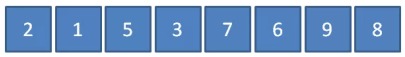• Hibbard增量（1, 3, 7, 15…2^k-1） —— 最坏时间复杂度是O(n^(3/2))
• Sedgewick增量（1, 5, 19, 41… 9*4k-9*2k+1或者4k-3*2k+1） —— 最坏时间复杂度是O(n^(4/3))
• Knuth增量（1, 4, 13, 40…1/2(3^k-1)） —— 最坏时间复杂度是O(n^(3/2))

### 5、归并排序（Merge Sort）

5.1 算法描述

• 把长度为 n 的输入序列分成两个长度为 n/2 的子序列；
• 对这两个子序列分别采用归并排序；
• 将两个排序好的子序列合并成一个最终的排序序列。

5.2 动图演示5.3 代码实现

 //归并排序
//需要temp存储合并后的值,合并的时候会有点麻烦
public static void mergeSort(int[] arr,int[] temp,int l,int r){
//涉及左右边界问题时，一定要注意边界交叉问题
if(l>=r) return;
int mid = (l+r)/2;

mergeSort(arr,temp,l,mid);

mergeSort(arr,temp,mid+1,r);

merge(arr,temp,l,r,mid);
}

private static void merge(int[] arr,int[] temp,int l ,int r,int mid ){
int i = l;
int j = mid+1;
//一定要注意这里是从0开始的
int index = 0;
//一定要注意这里是"<="的关系
while (i<=mid && j<=r){
if(arr[i]>=arr[j]){
temp[index++] = arr[j++];
}else{
temp[index++] = arr[i++];
}
}

while (i<=mid){
temp[index++] = arr[i++];
}

while (j<=r){
temp[index++] = arr[j++];
}
index = 0;
while (l<=r){
arr[l++] = temp[index++];
}
}



5. 4 算法分析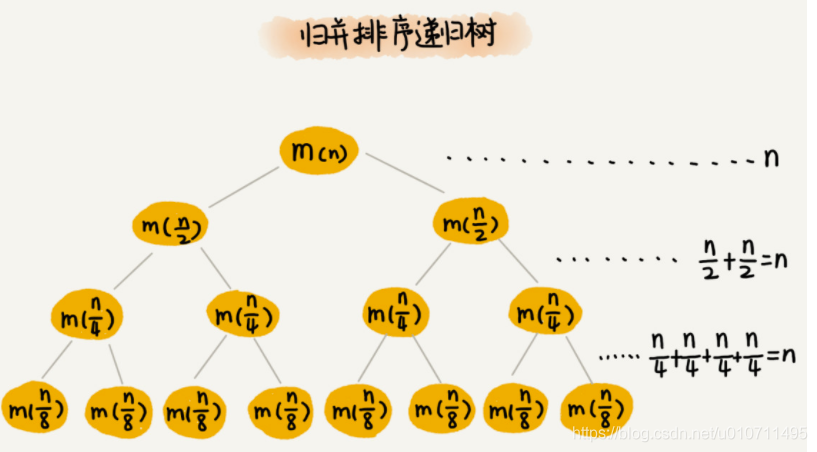### 6、快速排序（Quick Sort）

6.1 算法描述

• 从数列中挑出一个元素，称为 “基准”（pivot）；
• 重新排序数列，所有元素比基准值小的摆放在基准前面，所有元素比基准值大的摆在基准的后面（相同的数可以到任一边）。在这个分区退出之后，该基准就处于数列的中间位置。这个称为分区（partition）操作；
• 递归地（recursive）把小于基准值元素的子数列和大于基准值元素的子数列排序。

6.2 图解

1. 这里用R和L分别指向序列最右边和最左边。首先R开始向左移动，直到找到一个小于6的数停下来。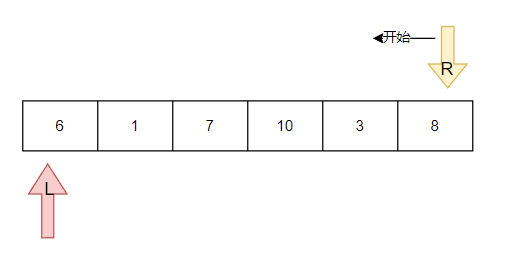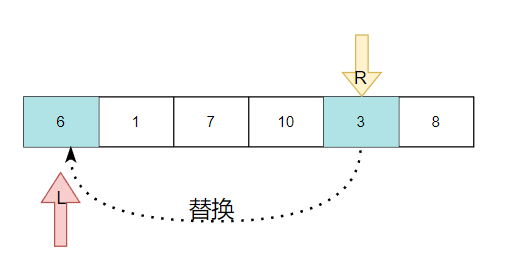1. 然后L开始向右移动，直到找到一个大于6的数停下来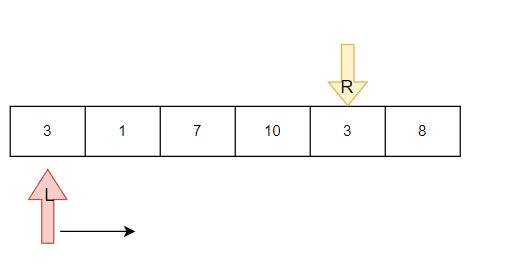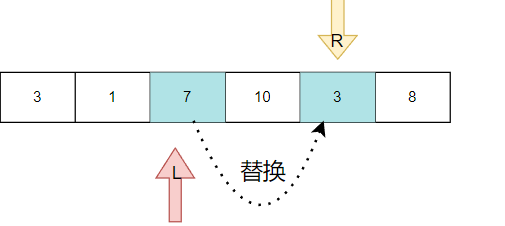1. 然后R开始向左移动，遇到L时停止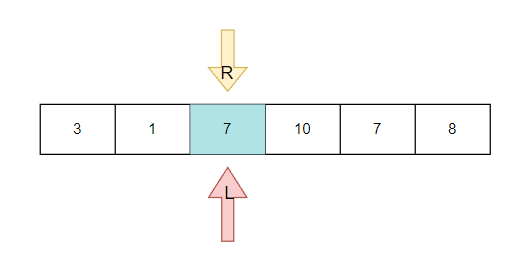1. 将L指向的数字替换为基准值temp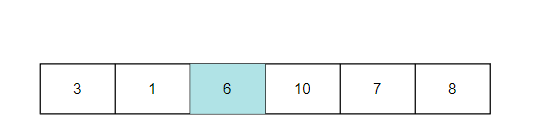5. 此时第一遍完成，然后把6左边的数和6右边的数再次进行快排

6.3 代码实现

//快排 考的概率很大，重点
public static void sort(int[] arr,int l,int r){
if (l>=r) return;
//优化,当长度较短时，使用插入排序
//        if(r-l<4){
//         这里使用插入排序
//           return;
//       }
int index = quickSort(arr, l, r);
sort(arr,l,index);
sort(arr,index+1,r);

}

public static int quickSort(int[] arr,int l,int r){
int temp = arr[l];
while (l<r){
while (l<r && arr[r]>=temp){
r--;
}
arr[l] = arr[r];
while (l<r&&arr[l]<=temp){
l++;
}
arr[r]=arr[l];
}
arr[l] = temp;
return l;
}


6.4 算法分析

0
1.2.• 0
1.2.